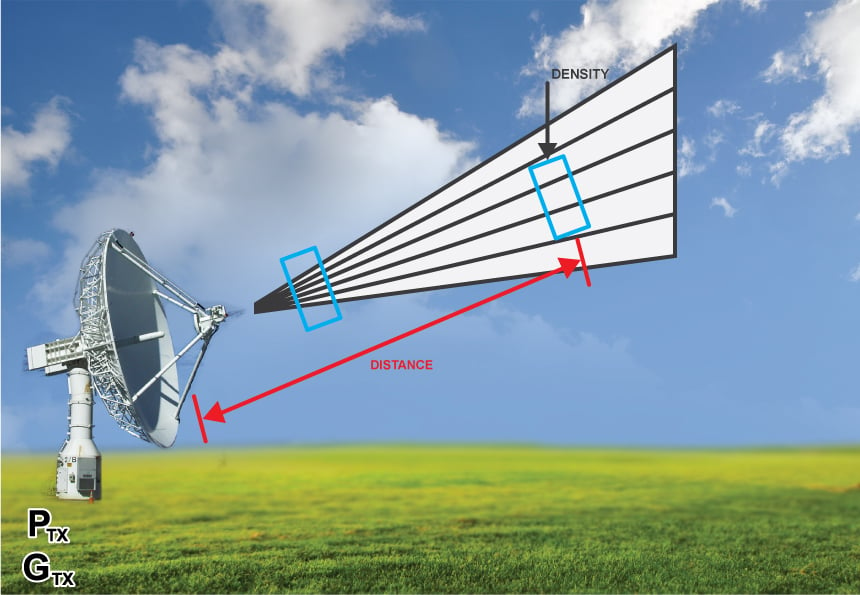# Power Density Calculator

## A tool designed to calculate the power density of an antenna

(linear)

(Watts/m2)

### OverviewPower density is the measure of the power from an antenna to a certain distance D. This assumes that an antenna radiates power in all directions. This calculator computes for the value of power density if the output power and gain are given.

### Equation

$$P_{D} = \frac{P_{out} G_{tx}}{4*\pi*D^{2}}$$

Where:

$$P_{D}$$ = power densit in watts/m2

$$P_{out}$$ = output power from the antenna in watts (W)

$$G_{tx}$$ = gain of the antenna

$$D$$ = distance from the antenna in meters (m)

### Applications

In antenna theory, an isotropic radiator is a theoretical, lossless antenna which radiates power uniformly in all directions. The power radiated from the isotropic antenna will have a uniform power per unit area no matter where you measure it from. The isotropic antenna therefore is the reference for all antennas. The power per unit area or power density of an isotropic radiator is:

$$P_{D} = \frac{P_{out}}{4*\pi*D^{2}}$$

You might have noticed that the only difference between the formulas for the power density of an isotropic radiator and that of any other antenna is the gain. This shows the need for calculating the power density of a real, practical antenna: it compares its power output to that of the reference isotropic antenna. Also, by knowing the power density, engineers can compute the power in any point from the antenna by simply multiplying PD to the capture area.